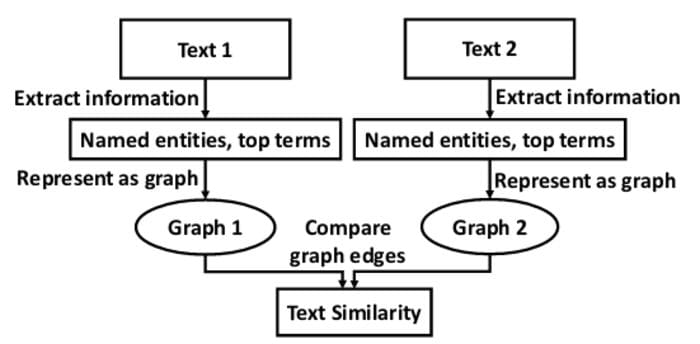# A Graph-based Text Similarity Method with Named Entity Information in NLP

In this article, the author summarizes the 2017 paper "A Graph-based Text Similarity Measure That Employs Named Entity Information" as per their understanding. Better understand the concepts by reading along.

By Prakhar Mishra, Research Scholar at IIIT-Bangalore

In this blog, I have tried summarizing the paper A Graph-based Text Similarity Measure That Employs Named Entity Information as per my understanding. Please feel free to comment your thoughts on the same!

### Problem Statement

The authors’ propose a novel technique for calculating Text similarity based on Named Entity enriched Graph representation of text documents. Objectively you can think of this as — Given two documents (D1, D2) we wish to return a similarity score (s) between them, where {s ∈ R|0 ≤ s ≤ 1} indicating the strength of similarity. 1 being exactly similar and 0 being dissimilar.

### Proposed MethodProposed pipeline | Image from Source

Authors’ propose a set of similarity measures over the n-gram graph representation for text documents. To do so, they propose a 3-step pipeline —

• Information Extraction — This is a first in the pipeline where they extract relevant information chunks from the text document for which they employ two methods: 1. Extraction of Named Entities 2. Extraction of Top-ranked terms using TF-IDF.
• Graph Representation — The information extracted from the first step is hashed (to get single node representation for multi-word terms) and used as unique nodes in the graph, whereas all the remaining words are replaced with a single placeholder word. Now, this is a modelling choice or you can think of it as a trade-off parameter to how many placeholder nodes would you want to represent. As the use of a single placeholder word results in a word graph having only one node for all the non-important words, which significantly reduces the size of the n-gram graph and the complexity of similarity operators. Let’s take an example to understand this — For instance, if the input sentence is “My name is Prakhar Mishra. I am a developer”. The pre-processed sentence representation becomes “A A A 213aaeb1 A A A _DEVELOPER”, where, is the placeholder symbol for unimportant words, 213aaeb1 is the hash for Prakhar Mishra and _DEVELOPER is the hash for the word developer. Refer to the below figure to understand this visually —N-gram Graph Representation

The edges are weights that you see in the above n-gram graph are decided based on the co-occurrence count of terms in a sliding window of size L traversing over the pre-processed sentence representation.

• Graph Similarity Measures — Once we have the graph ready, the authors’ employ metrics such as Value SimilaritySize Similarity and Normalized Value Similarity for measuring the similarity between the two graphs, where,

— Value Similarity: This takes into account the set of common edges between two graphs along with their respective weights. It is mathematically represented as:value similarity

where, e is the common edge between two graphs Gi, Gj and VR(e) is calculated as:VR calculation

— Size Similarity: It takes into account the size of the graphs, which is calculated as:size similarity

— Normalized Value Similarity: This similarity measure ignores the relative size of the graph during comparison. And is defined as:normalized value similarity

If SS (Size similarity)=0, then value of NVS is also set to Zero.

Depending on the use case one can decide on how to use the above set of similarity measures. We can merge the scores from all the above methods using some pooling function and represent it as an aggregated similarity score. Also, another way is to represent the graph as a vector of similarity scores from the above methods and then perform clustering or classification on-top.

### Possible Extensions (My thoughts)

We can have a little controlled way of hashing wherein the same hash is given to the same entity groups. As this would inducing categorical similarity in the graph and would also reduce the space/time complexity.

You can also checkout other research paper explanations that i have written —

10 Popular Keyword Extraction Algorithms in NLP

BERT-QE: Contextualized Query Expansion

Beyond Accuracy: Behavioral Testing of NLP Models using CheckList

BERT for Extractive Text Summarization

Feel free to read the paper and say “Hi” to the authors and appreciate their contribution.

Paper Title: A Graph-based Text Similarity Measure That Employs Named Entity Information

Paper Link: Access Paper

Thank you!

Bio: Prakhar Mishra Prakhar is currently a MS (by research) grad student in Data Science at IIIT Bangalore. His research interest include Natural Language Understanding and Generation, Information Retrieval, Unsupervised Machine Learning and Reinforcement Learning.

Original. Reposted with permission.

Related:

By subscribing you accept KDnuggets Privacy Policy

By subscribing you accept KDnuggets Privacy Policy

By subscribing you accept KDnuggets Privacy Policy